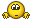We use cookies to ensure that we give you the best experience on our website. If you continue to use this site we will assume that you are happy with it.

# Generating procedural noise in V3d

Home Forums Graphics / Maya Generating procedural noise in V3d

Viewing 6 posts - 1 through 6 (of 6 total)
• Author
Posts
• #38021

Hi guys

this might be a noob question, but how can you make a procedural noise with the noise node not yet supported in Verge3D for Maya? I tried the OSL but it doesn’t seem to work on iOS#38261

Unfortunally, there’s no procedural noises supported for Maya yet.

Co-founder and lead graphics specialist at Soft8Soft.

#38292

Hi, what kind of OSL shader you tried? Could you please provide the code listing here?

Verge3D’s Tech Chief

#38344

This one, It’s the 3ds Max’s osl noise

#38345

weired… it doesn’t want to upload the file

``````// General Noise Shader, returning a float (1D)
// Modified: 2019-11-26

(
point  UVW    = transform("object", P)
[[ string help = "The UVW coordinate to use. When not connected, defaults to Object space" ]],
float  Scale  = 25.0,
string Type   = "uperlin"
[[ string widget= "popup",
string help  = "Use perlin, uperlin, cell, hash, simplex or gabor",
string options="perlin|uperlin|cell|hash|simplex|gabor" ]],
int   Octaves    = 4
[[ string help = "Hos many layers of noise are mixed together" ]],
float Lacunarity = 2.0
[[ string help = "How much the 'frequency' of the noise changes per layer" ]],
float Gain       = 0.5
[[ string help = "How much the amplitude of the noise changes per layer. Higher numbers means higher noise frequencies have more effect." ]],

int   StepFunction = 1
[[ string widget= "checkBox",
string label = "Step Function",
string help  = "Enables a per-layer smoothstep curve in the noise, allowing you to increase the 'contrast' of the noise" ]],
float LowStep = 0.5
[[ string help = "Low threshold of the smoothstep function.",
string label = "Low Step",
float  min = -1.0, float max = 1.0 ]],
float HiStep = 0.8
[[ string help = "High threshold of the smoothstep function.",
string label = "High Step",
float  min = -1.0, float max = 1.0 ]],
int   Normalize = 1
[[ string widget= "checkBox",
string help = "If the noise is auto-normalized to Amplitude or not." ]],
float Amplitude = 1.0
[[ string help = "The amplitude of the noise." ]],
float Phase     = 0.0
[[ string help = "The 'Phase' is just a 4th coordinate of the noise, can be used to allow it to evolve over time, for example." ]],

output float Out = 0,
)
{
point pnt = UVW / Scale;

float sum = 0;
float curFreq = 1.0;
float curAmp = Amplitude;

// Loop over number of octaves
for (int i = 0; i < Octaves; i++)
{
// Compute a noise value
float ns = noise(Type, pnt * curFreq, Phase + i);

if (StepFunction)
ns = smoothstep(LowStep, HiStep, ns);

// Add our result to the output
Out += ns * curAmp;
// Add the amplitude to the normalizing sum
sum += curAmp;

// Step up frequency and amplitude
curFreq *= Lacunarity;
curAmp  *= Gain;
}

if (Normalize)
Out /= sum / Amplitude;
}
``````
#39208

Hi,
WebGL 1.0 (which is the only version supported on iOS devices now) can’t handle loops with non-constant comparisons. Try this code instead:

``````// General Noise Shader, returning a float (1D)
// Modified: 2019-11-26

(
point  UVW    = transform("object", P)
[[ string help = "The UVW coordinate to use. When not connected, defaults to Object space" ]],
float  Scale  = 25.0,
string Type   = "uperlin"
[[ string widget= "popup",
string help  = "Use perlin, uperlin, cell, hash, simplex or gabor",
string options="perlin|uperlin|cell|hash|simplex|gabor" ]],
int   Octaves    = 4
[[ string help = "Hos many layers of noise are mixed together" ]],
float Lacunarity = 2.0
[[ string help = "How much the 'frequency' of the noise changes per layer" ]],
float Gain       = 0.5
[[ string help = "How much the amplitude of the noise changes per layer. Higher numbers means higher noise frequencies have more effect." ]],

int   StepFunction = 1
[[ string widget= "checkBox",
string label = "Step Function",
string help  = "Enables a per-layer smoothstep curve in the noise, allowing you to increase the 'contrast' of the noise" ]],
float LowStep = 0.5
[[ string help = "Low threshold of the smoothstep function.",
string label = "Low Step",
float  min = -1.0, float max = 1.0 ]],
float HiStep = 0.8
[[ string help = "High threshold of the smoothstep function.",
string label = "High Step",
float  min = -1.0, float max = 1.0 ]],
int   Normalize = 1
[[ string widget= "checkBox",
string help = "If the noise is auto-normalized to Amplitude or not." ]],
float Amplitude = 1.0
[[ string help = "The amplitude of the noise." ]],
float Phase     = 0.0
[[ string help = "The 'Phase' is just a 4th coordinate of the noise, can be used to allow it to evolve over time, for example." ]],

output float Out = 0,
)
{
point pnt = UVW / Scale;

float sum = 0;
float curFreq = 1.0;
float curAmp = Amplitude;

// Loop over number of octaves
for (int i = 0; i < 4; i++)
{
// Compute a noise value
float ns = noise(Type, pnt * curFreq, Phase + i);

if (StepFunction)
ns = smoothstep(LowStep, HiStep, ns);

// Add our result to the output
Out += ns * curAmp;
// Add the amplitude to the normalizing sum
sum += curAmp;

// Step up frequency and amplitude
curFreq *= Lacunarity;
curAmp  *= Gain;
}

if (Normalize)
Out /= sum / Amplitude;
}
``````

Also, we’re working on supporting noise shader in Maya. Check out the upcoming Verge3D preview release.

Verge3D’s Tech Chief